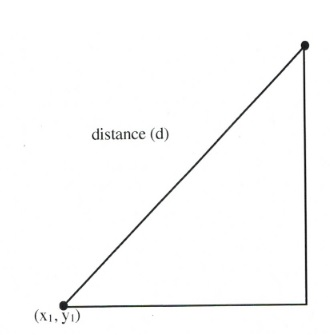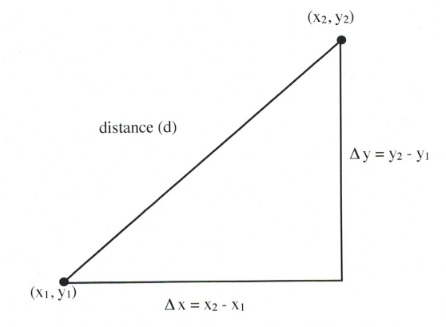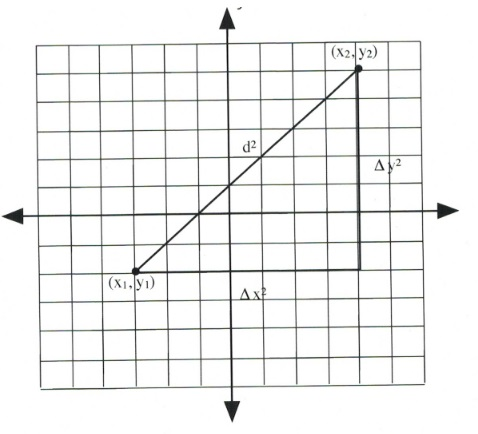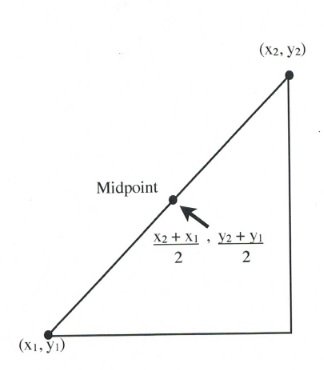Chapter 3: Graphing

# Finding the Distance Between Two Points

The logic used to find the distance between two data points on a graph involves the construction of a right triangle using the two data points and the Pythagorean theorem $(a^2 + b^2 = c^2)$ to find the distance.

To do this for the two data points $(x_1, y_1)$ and $(x_2, y_2)$, the distance between these two points $(d)$ will be found using $\Delta x = x_2 - x_1$ and $\Delta y = y_2 - y_1.$

Using the Pythagorean theorem, this will end up looking like:

$d^2 = \Delta x^2 + \Delta y^2$or, in expanded form:

$d^2 = (x_2 - x_1)^2 + (y_2 - y_1)^2$On graph paper, this looks like the following. For this illustration, both $\Delta x$ and $\Delta y$ are 7 units long, making the distance $d^2 = 7^2 + 7^2$ or $d^2 = 98$.The square root of 98 is approximately 9.899 units long.

Example 3.2.1

Find the distance between the points $(-6,-4)$ and $(6, 5)$.

Start by identifying which are the two data points $(x_1, y_1)$ and $(x_2, y_2)$. Let $(x_1, y_1)$ be $(-6,-4)$ and $(x_2, y_2)$ be $(6, 5)$.

Now:

$\Delta x^2 = (x_2 - x_1)^2$ or $[6 - (-6)]^2$ and $\Delta y^2 = (y_2 - y_1)^2$ or $[5 - (-4)]^2$.

This means that

$d^2 = [6 - (-6)]^2 + [5 - (-4)]^2$

or

$d^2 = ^2 + ^2$

which reduces to

$d^2 = 144 + 81$

or

$d^2 = 225$

Taking the square root, the result is $d = 15$.

# Finding the Midway Between Two Points (Midpoint)

The logic used to find the midpoint between two data points $(x_1, y_1)$ and $(x_2, y_2)$ on a graph involves finding the average values of the $x$ data points $(x_1, x_2)$ and the of the $y$ data points $(y_1, y_2)$. The averages are found by adding both data points together and dividing them by $2$.

In an equation, this looks like:

$x_{\text{mid}}=\dfrac{x_2+x_1}{2}$ and $y_{\text{mid}}=\dfrac{y_2+y_1}{2}$

Example 3.2.2

Find the midpoint between the points $(-2, 3)$ and $(6, 9)$.We start by adding the two $x$ data points $(x_1 + x_2)$ and then dividing this result by 2.

$x_{\text{mid}} = \dfrac{(-2 + 6)}{2}$

or

$\dfrac{4}{2} = 2$

The midpoint’s $y$-coordinate is found by adding the two $y$ data points $(y_1 + y_2)$ and then dividing this result by 2.

$y_{\text{mid}} = \dfrac{(9 + 3)}{2}$

or

$\dfrac{12}{2} = 6$

The midpoint between the points $(-2, 3)$ and $(6, 9)$ is at the data point $(2, 6)$.

# Questions

For questions 1 to 8, find the distance between the points.

1.  (−6, −1) and (6, 4)
2. (1, −4) and (5, −1)
3. (−5, −1) and (3, 5)
4. (6, −4) and (12, 4)
5. (−8, −2) and (4, 3)
6. (3, −2) and (7, 1)
7. (−10, −6) and (−2, 0)
8. (8, −2) and (14, 6)

For questions 9 to 16, find the midpoint between the points.

1. (−6, −1) and (6, 5)
2. (1, −4) and (5, −2)
3. (−5, −1) and (3, 5)
4. (6, −4) and (12, 4)
5. (−8, −1) and (6, 7)
6. (1, −6) and (3, −2)
7. (−7, −1) and (3, 9)
8. (2, −2) and (12, 4)